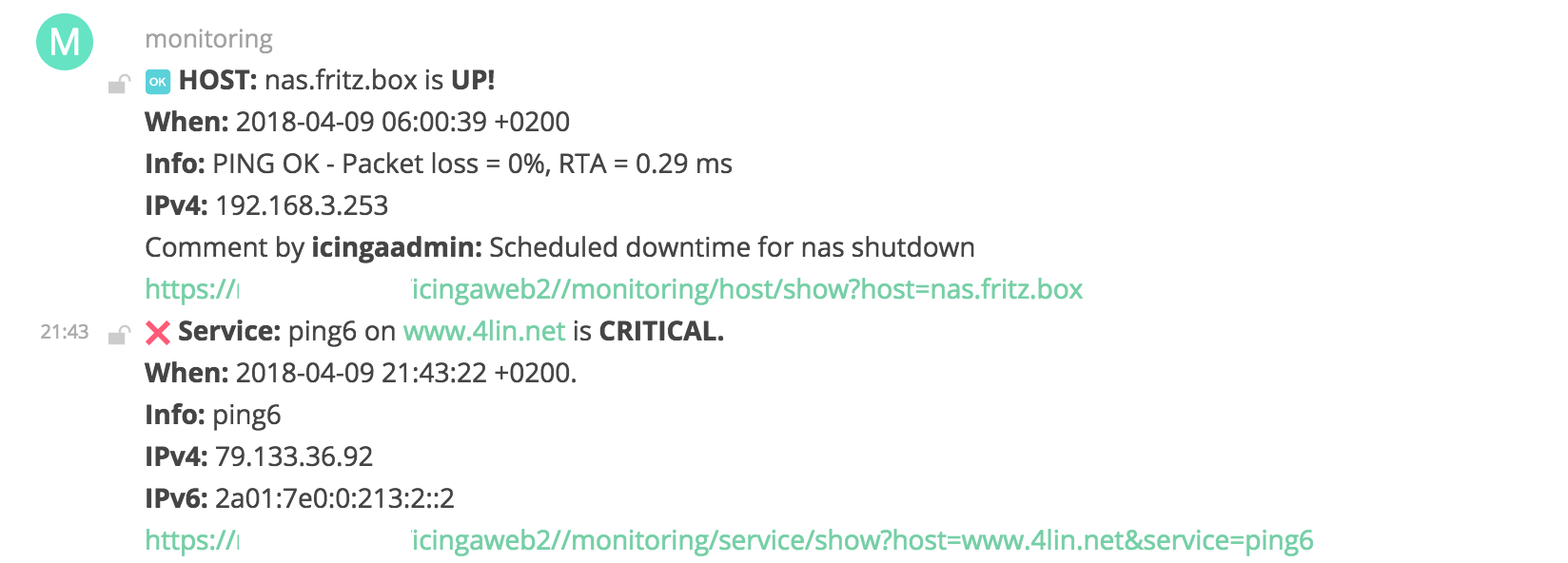Icinga2 notification to Matrix.org chat rooms

Sent Icinga2 notifications to Matrix.org or own Matrix homeserver chatroom

The scripts itself are just a bad clone from the original mail notifications, which are from https://github.com/sysadmama if I remember correctly. The "only" difference is -- that instead of creating a mail -- we use curl to submit the notification into a room.To send notifications from Icinga2 into a room, the following conditions are required:

• A matrix compatible server, e.g matrix-synapse or use https://matrix.org
• A Matrix room ID

There exists several ways for creating a Matrix user, but an easy way is to create a new user (for example "monitoring" via login in Riot web); get the access token and invite the new Matrix user into the room, which may was created for the monitoring. A good approach is to have several rooms, for example devops, sysops, web, dba .... and use the apply rule to assign the correct room with the \$notification_matrix_room_id\$ .

The following configuration examples should work in most cases :-)

## commands.cfg

``````object NotificationCommand "Host Alarm by Matrix" {
command = [ SysconfDir + "/icinga2/scripts/matrix-host-notification.sh" ]
arguments += {
"-d" = {
required = true
}
"-l" = {
required = true
}
"-m" = {
required = true
}
"-n" = {
required = true
}
"-o" = {
required = true
}
"-s" = {
required = true
}
"-t" = {
required = true
}
"-x" = {
required = true
}
"-y" = {
required = true
}
}
}``````
``````object NotificationCommand "Service Alarm by Matrix" {
command = [ SysconfDir + "/icinga2/scripts/matrix-service-notification.sh" ]
arguments += {
"-4" = {
required = true
}
"-d" = {
required = true
}
"-e" = {
required = true
}
"-l" = {
required = true
}
"-m" = {
required = true
}
"-n" = {
required = true
}
"-o" = {
required = true
}
"-s" = {
required = true
}
"-t" = {
required = true
}
"-u" = {
required = true
}
"-x" = {
required = true
}
"-y" = {
required = true
}
}

## services.cfg

``````/**
* Example Matrix.org apply rules.
* The "!:matrix.org" needs to be replaced with the room ID
* for example "!SDFfskjfdszhdaslasdkjhdasd:matrix.org".
*/

apply Notification "Matrix host problems" to Host {

}

apply Notification "Matrix service problems" to Service {

}``````

## templates.cfg

``````/**
* Provides default settings for Matrix.org service notifications.
*/

states = [ Up, Down ]
types = [ Problem, Acknowledgement, Recovery, Custom,
FlappingStart, FlappingEnd,
DowntimeStart, DowntimeEnd, DowntimeRemoved ]
vars += {
}
// interval = 0s
period = "24x7"
}

states = [ OK, Warning, Critical, Unknown ]
types = [ Problem, Acknowledgement, Recovery, Custom,
FlappingStart, FlappingEnd,
DowntimeStart, DowntimeEnd, DowntimeRemoved ]

vars += {